Chapter 3 Stoichiometry Calculations with Chemical Formulas Equations

• Slides: 18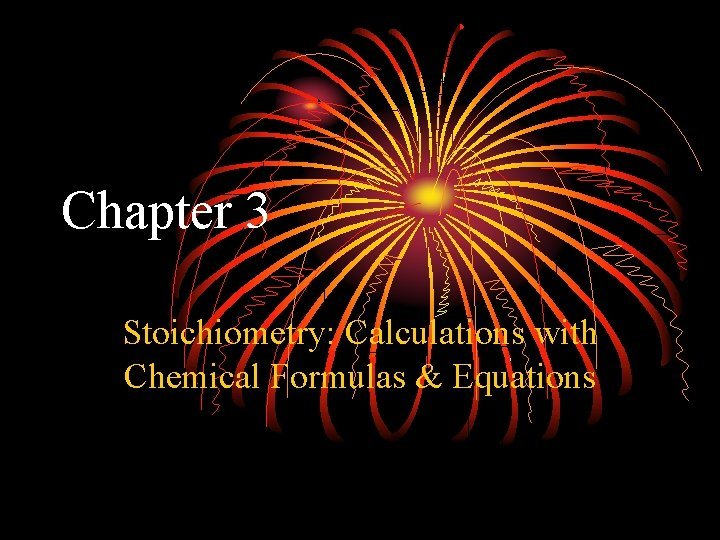Chapter 3 Stoichiometry: Calculations with Chemical Formulas & EquationsStoichiometry & Chemical Eq’ns Stoich: the study of the rearrangement of atoms in molecules during chemical rxns Chem eq’n: the written expression of a chemical process Subscripts: denote the number of each atom in a chemical formula; used to balance charge; are not changed when balancing rxns Coefficients: denote the number of molecules in a rxn; may be changed to balance a rxn; demonstrate LOCOM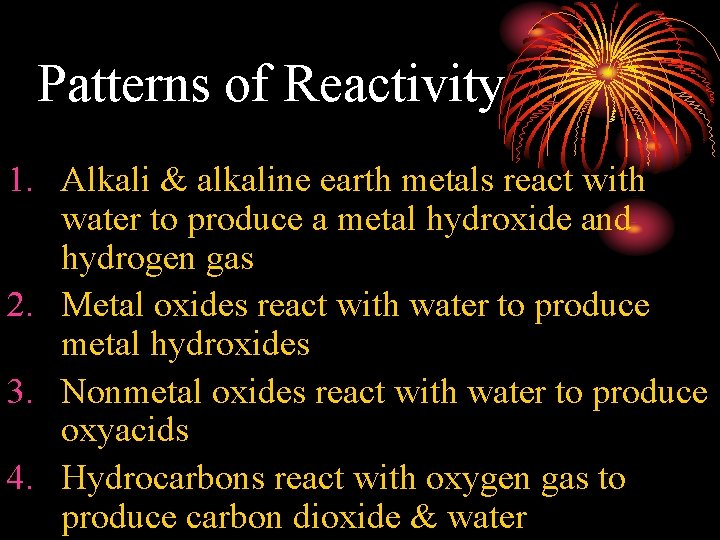Patterns of Reactivity 1. Alkali & alkaline earth metals react with water to produce a metal hydroxide and hydrogen gas 2. Metal oxides react with water to produce metal hydroxides 3. Nonmetal oxides react with water to produce oxyacids 4. Hydrocarbons react with oxygen gas to produce carbon dioxide & water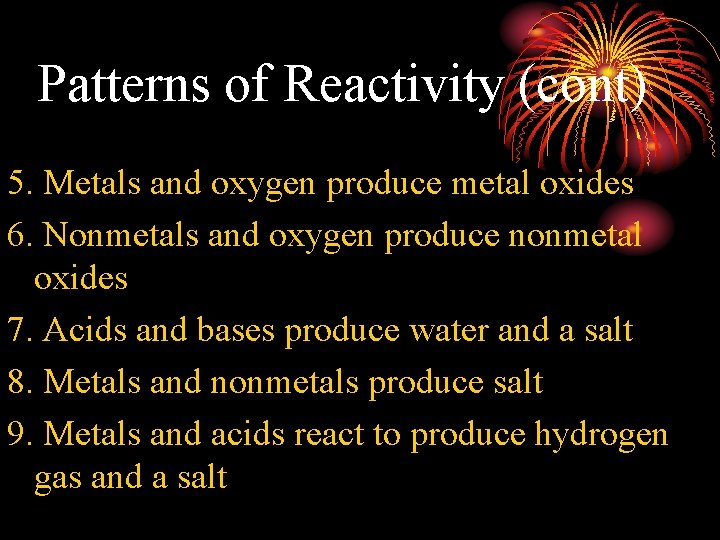Patterns of Reactivity (cont) 5. Metals and oxygen produce metal oxides 6. Nonmetals and oxygen produce nonmetal oxides 7. Acids and bases produce water and a salt 8. Metals and nonmetals produce salt 9. Metals and acids react to produce hydrogen gas and a salt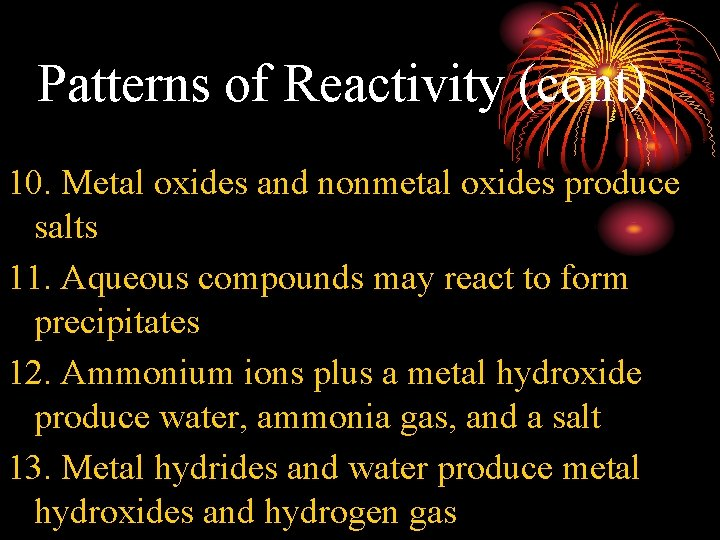Patterns of Reactivity (cont) 10. Metal oxides and nonmetal oxides produce salts 11. Aqueous compounds may react to form precipitates 12. Ammonium ions plus a metal hydroxide produce water, ammonia gas, and a salt 13. Metal hydrides and water produce metal hydroxides and hydrogen gas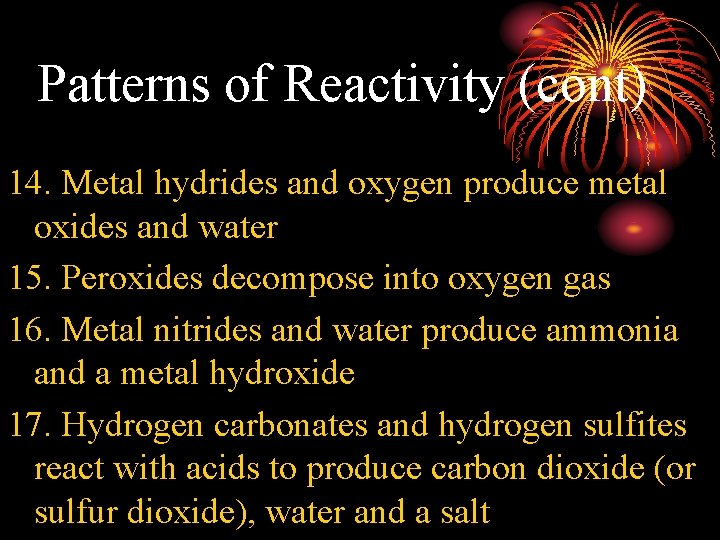Patterns of Reactivity (cont) 14. Metal hydrides and oxygen produce metal oxides and water 15. Peroxides decompose into oxygen gas 16. Metal nitrides and water produce ammonia and a metal hydroxide 17. Hydrogen carbonates and hydrogen sulfites react with acids to produce carbon dioxide (or sulfur dioxide), water and a saltPatterns of Reactivity (cont) 18. Superoxides react with water to produce oxygen gas, hydrogen peroxide, and aqueous alkali and hydroxide ions 19. Metal carbides and water produce acetylene (C 2 H 2 - explosive) and a metal hydroxide 20. Ammonia and hypochlorite ions react to produce water, salt, and hydrazine gas (N 2 H 4 deadly poisonous)Atomic & Molecular Weights 1 amu = 1. 66054 x 10 -24 g 1 g = 6. 02214 x 1023 amu Average atomic mass: the weighted average of all naturally occurring isotopes Formula weight: sum of the average atomic masses of all elements in the compound; also known as molecular weight for molecular substances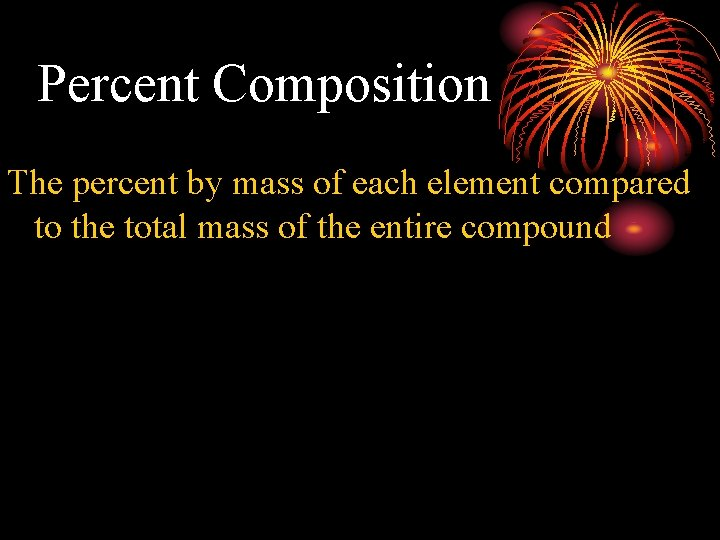Percent Composition The percent by mass of each element compared to the total mass of the entire compound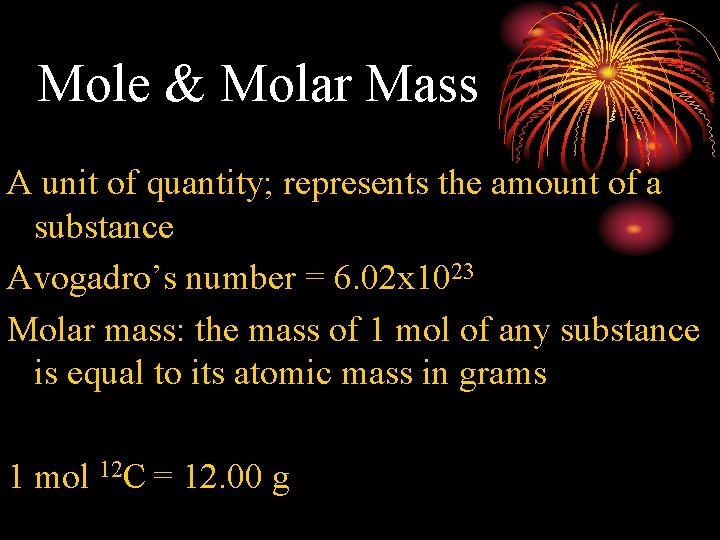Mole & Molar Mass A unit of quantity; represents the amount of a substance Avogadro’s number = 6. 02 x 1023 Molar mass: the mass of 1 mol of any substance is equal to its atomic mass in grams 1 mol 12 C = 12. 00 g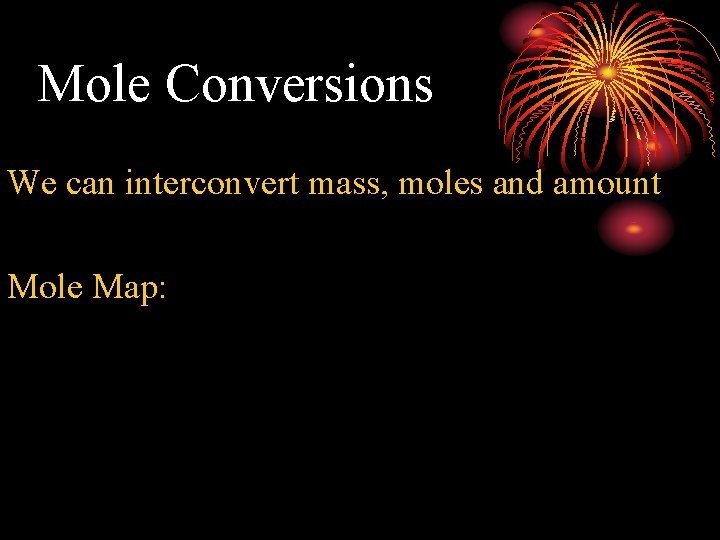Mole Conversions We can interconvert mass, moles and amount Mole Map: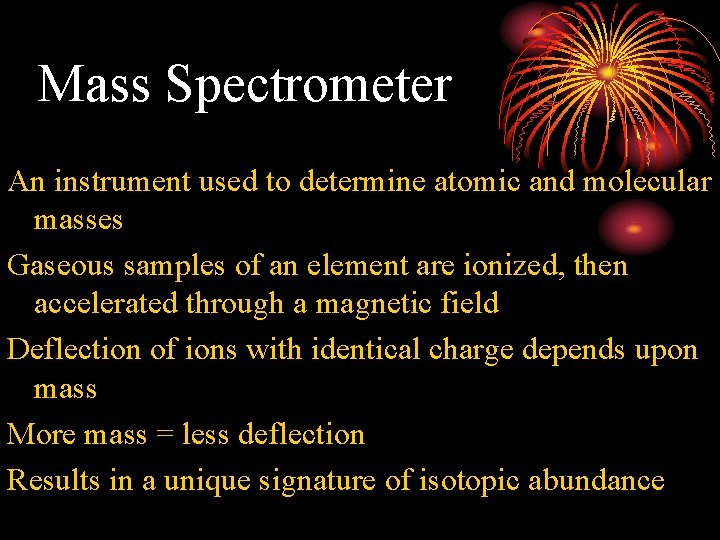Mass Spectrometer An instrument used to determine atomic and molecular masses Gaseous samples of an element are ionized, then accelerated through a magnetic field Deflection of ions with identical charge depends upon mass More mass = less deflection Results in a unique signature of isotopic abundance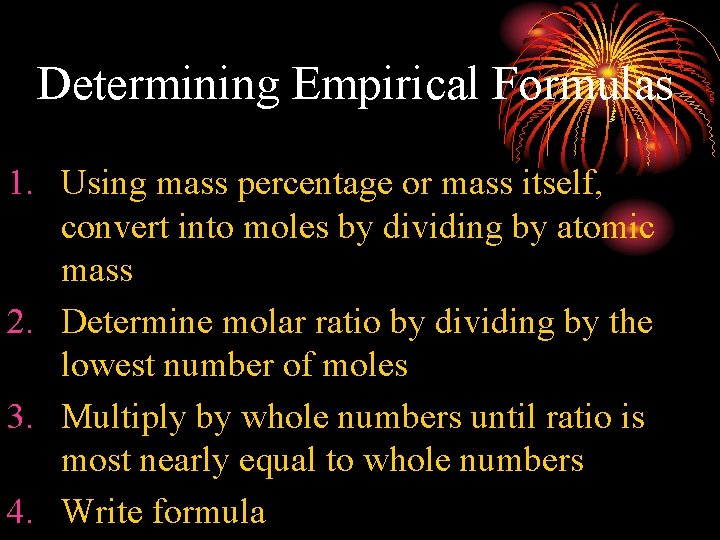Determining Empirical Formulas 1. Using mass percentage or mass itself, convert into moles by dividing by atomic mass 2. Determine molar ratio by dividing by the lowest number of moles 3. Multiply by whole numbers until ratio is most nearly equal to whole numbers 4. Write formula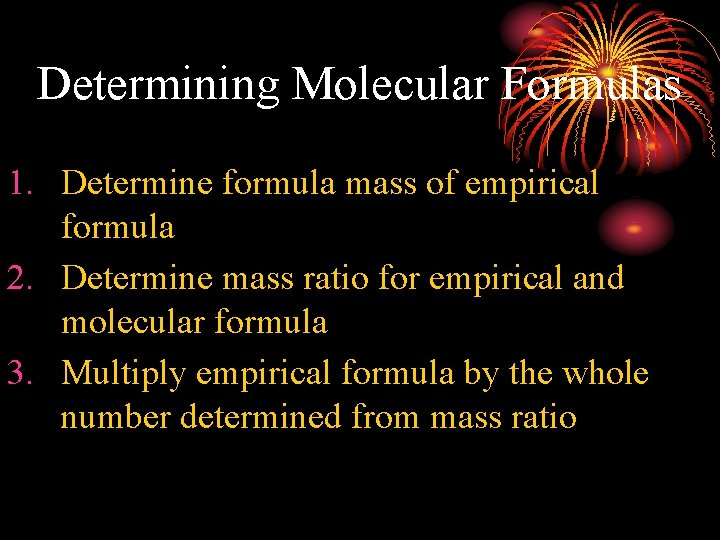Determining Molecular Formulas 1. Determine formula mass of empirical formula 2. Determine mass ratio for empirical and molecular formula 3. Multiply empirical formula by the whole number determined from mass ratioCombustion Analysis Many samples are determined through combustion analysis 1. Determine moles of C and H first 2. Subtract from initial mass to determine moles of O 3. Determine molar ratios and write formula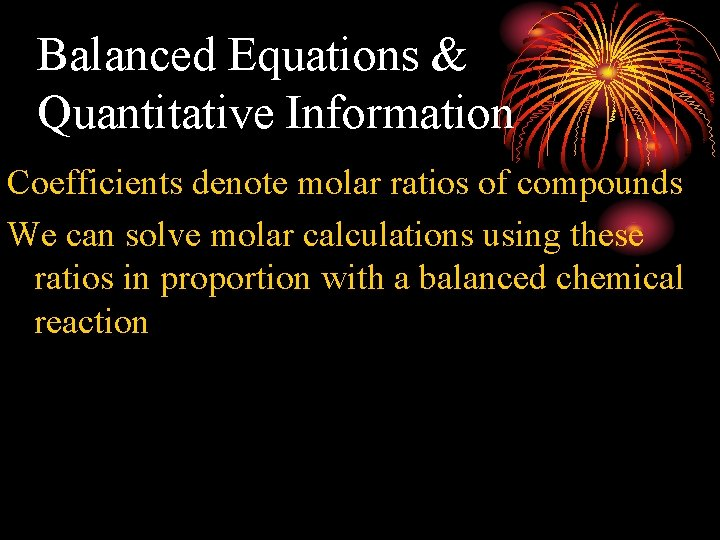Balanced Equations & Quantitative Information Coefficients denote molar ratios of compounds We can solve molar calculations using these ratios in proportion with a balanced chemical reactionLimiting Reactant The reactant in a chemical process that is entirely consumed; determines the amount of product made in the reaction Any reactant could be the limiting reactant - it depends on a particular set of conditionsTheoretical, Actual, & % Yield The amount of product one expects based upon the molar ratios and amounts of reactants involved in the chemical process Actual Yield – the amount of product recovered in the experiment Percent Yield = actual/theoretical x 100